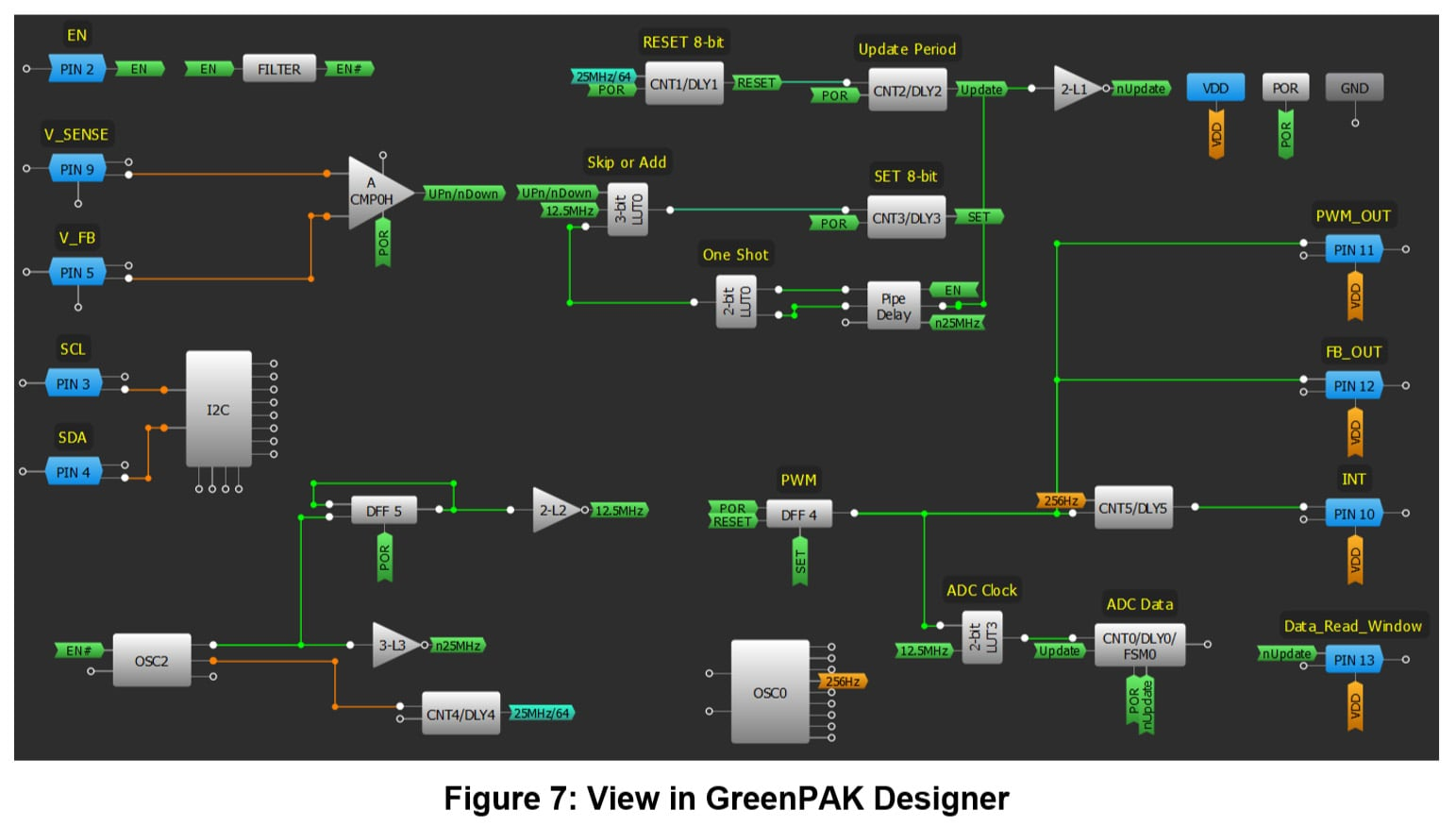CustomApril 14, 2021 by GreenPAK™ Family of Integrated Circuits
Share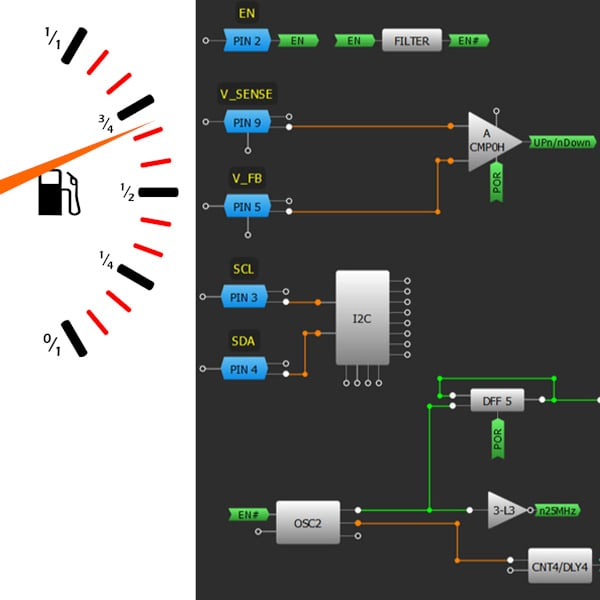Check outhow to implement an analog-to-digital converter (ADC) in the SLG46855V to measure current drawn by a load

## Hardware

Below we described steps needed to understand how the solution has been programmed to create the ADC current sense. However, if you just want to get the result of programming, download GreenPAKâ„¢ Designer software to view the already completed GreenPAK Design File. Plug the GreenPAK Development Kit to your computer and hit the program to design the device.

In this project, we will describe how to implement an 8-bit analog-to-digital converter (ADC) in the SLG46855V that can sense load current and interface with an MCU via I2C. This design can be used for various current sensing applications such as ammeters, fault detection systems, and fuel gauges.

The ADC is essentially comprised of an analog comparator and a Digital-to-Analog Converter (DAC). The comparator senses the input voltage vs. the DAC output voltage and subsequently controls whether to increment or decrement the DAC input code, such that the DAC output converges to the input voltage. The resulting DAC input code becomes the ADC digital output code.

In our implementation, we create a DAC using pulse-width modulation (PWM) controlled resistor network. We can easily create a precise digitally controlled PWM output using GreenPAK. The PWM when filtered becomes our analog voltage and thus serves as an effective DAC. A distinct advantage of this approach is that it is easy to set the voltages which corresponds to zero code and full scale (equivalently offset and gain) by simply adjusting resistor values. For example, a user wants to ideally read zero code from a temperature sensor with no current (0 ÂµA) corresponding to 4.3 V, and full-scale code at 1000 ÂµA corresponding to 3.9 V (Table 1). This is easily implemented by simply setting a few resistor values. By having the ADC range match the sensor range of interest, we make the greatest use of the ADC resolution.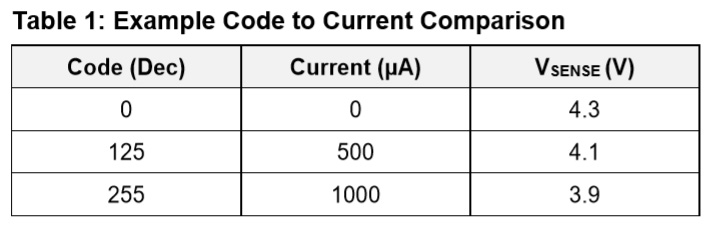A design consideration for this architecture is that an internal PWM frequency needs to be much faster than the ADC update rate to prevent underdamped behavior of its control loop. At the very least it should be longer than the ADC data counter clock divided by 256. In this design, the ADC update period is set to 1.3312 ms.

## Internal Circuit

The flexible ADC is based on the design presented in Dialog Semiconductor AN-1177. The clock speed is increased from 1 MHz to 12.5 MHz in order to clock the ADC counter since the SLG46855 has a 25 MHz clock available. This allows a much faster update rate for finer sample resolution. The LUT clocking the ADC data clock is changed so it will pass through the 12.5 MHz signal when the PWM DFF is low.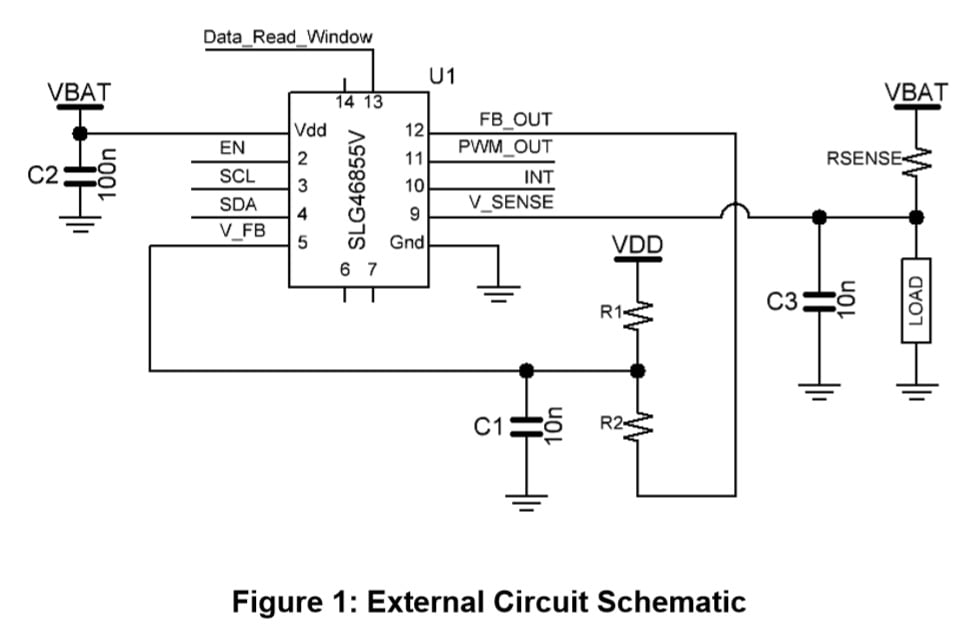## External Circuit

An external resistor and capacitor network are used to convert a PWM into an analog voltage as shown in the circuit schematic in Figure 1. The values are calculated for maximum resolution for the maximum current the device will sense. To achieve this flexibility, we add resistors R1 and R2 in parallel to VDD and ground. A resistor divider divides down VBAT to the low side of the voltage range. The divider ratio for an expected minimum VBAT can be solved using the following equation:Table 1 describes the I2C command structure to read back the data stored in CNT0. The I2C commands require a start bit, control byte, word address, read bit, and stop bit.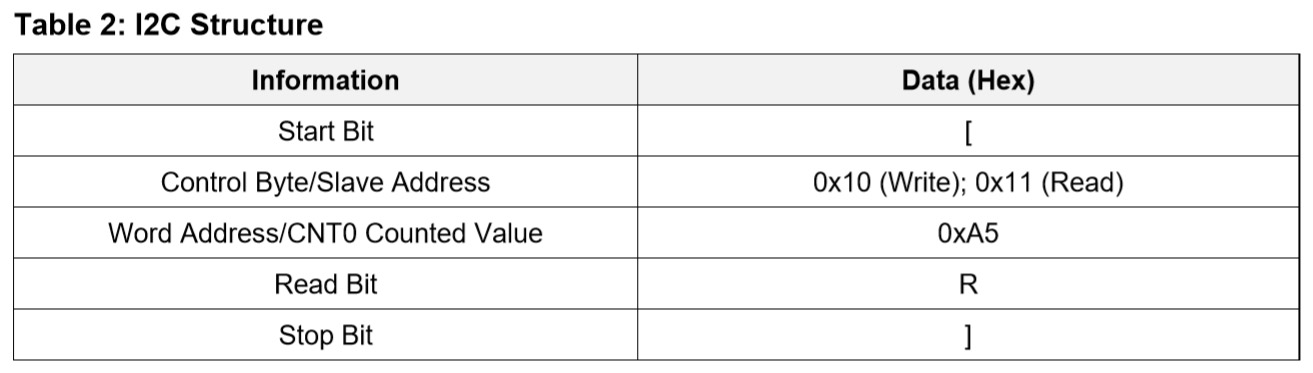An example I2C command to read back the CNT0 counted value is written below:

[0x10 0xA5] [0x11 R]

The counted value that is read back will be the ADC code value. As an example, an Arduino code is included in the ZIP file of this project on Dialog's website.

## Results

To test the accuracy of the ADC current sense design, the measured values at a given load current and VDD level were compared to a theoretical value. The theoretical ADC values were calculated with Equation 2. The ILOAD that correlates with an ADC value is found with Equation 3.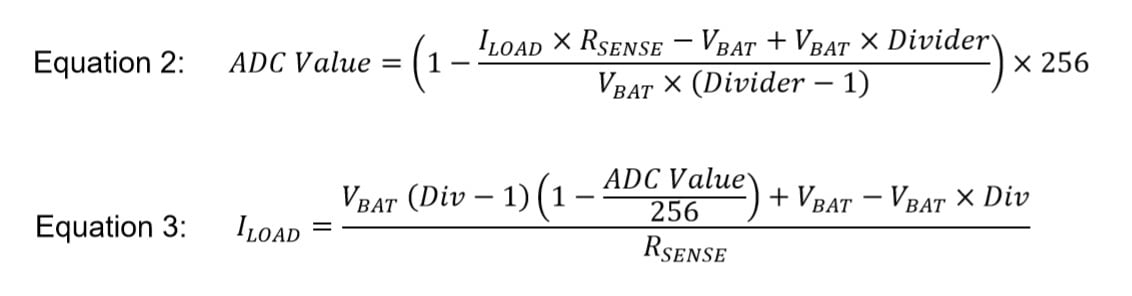For the following results I used these component values: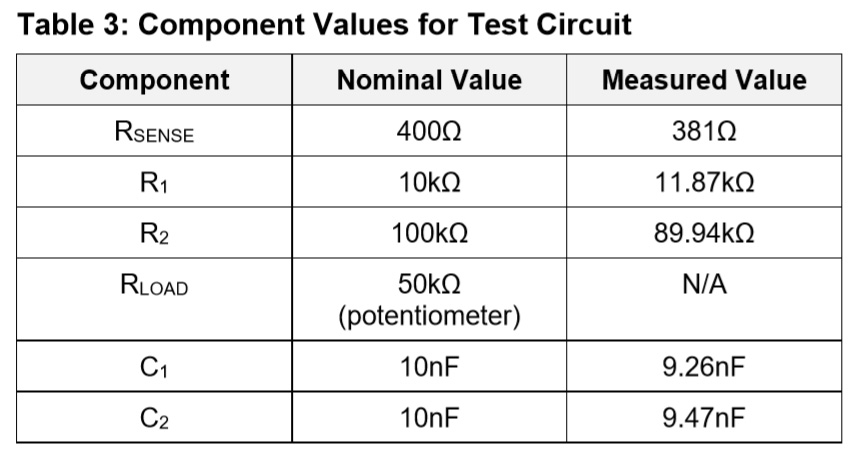The resolution of the ADC value to ILOAD conversion can be calculated by using equation 3 with the measured values in Table 2 and the ADC value set to 1. With a VBAT of 3.9 V the resolution is 4.96 ÂµA/div.

In order to optimize the ADC current sense circuit to a minimum VDD level of 3.6 V with a maximum current of 1100 ÂµA and a 381 Î© sense resistor, the ideal divider coefficient would be 0.884, based on equation 1. With the values given in Table 2, the actual divider has a divider coefficient of 0.876. Since this is slightly less, it will allow for a slightly larger load current range so the ADC values are close to the full range but will not overflow. The actual divider value is calculated with the following equation:Below are the measurements taken of the circuit at three voltage levels: 4.3 V, 3.9 V, and 3.6 V. Each level displays a graph displaying the difference between the measured and theoretical ADC values. Theoretical values are rounded to the closest whole integer. There is a summary graph to compare the differences at the three voltage levels. Afterwards there is a graph displaying the correlation between the theoretical ADC values and load current at the different voltage levels.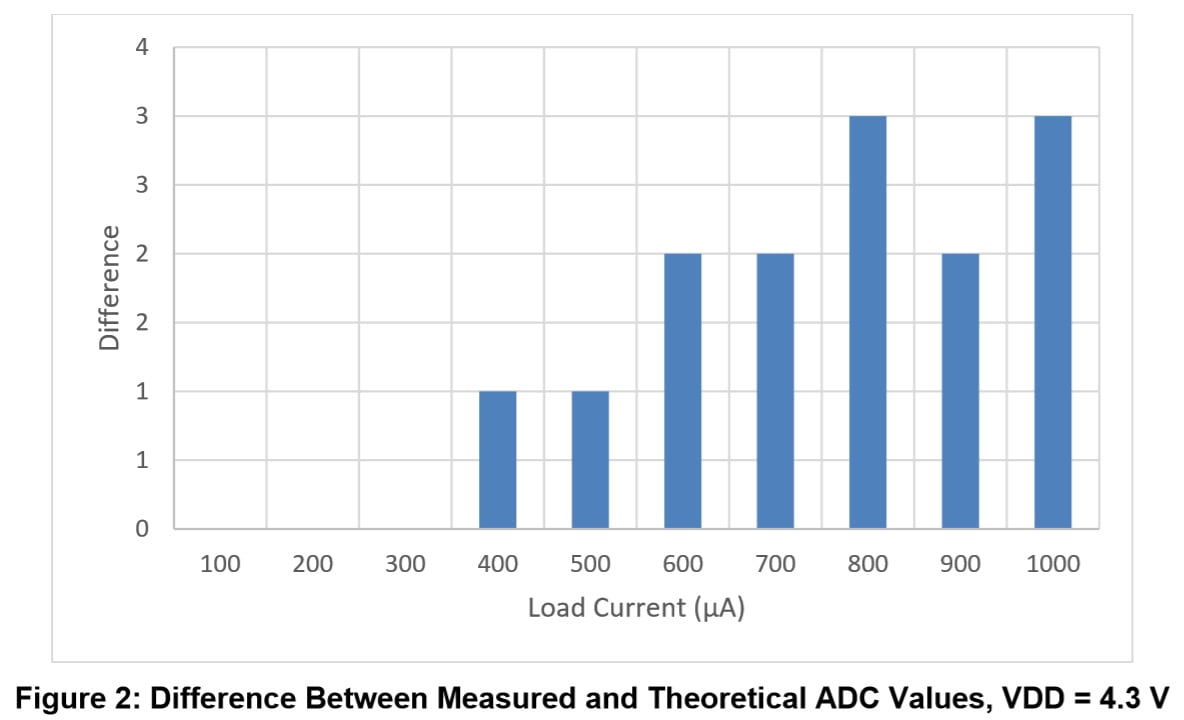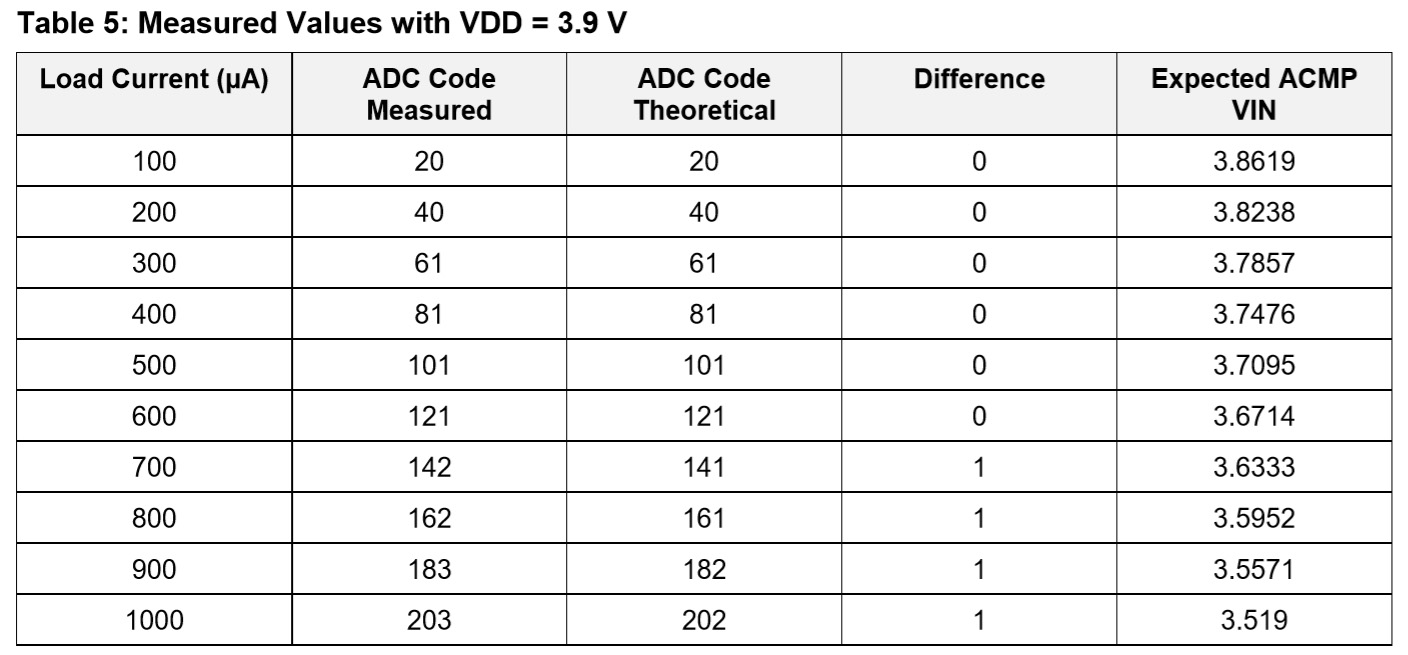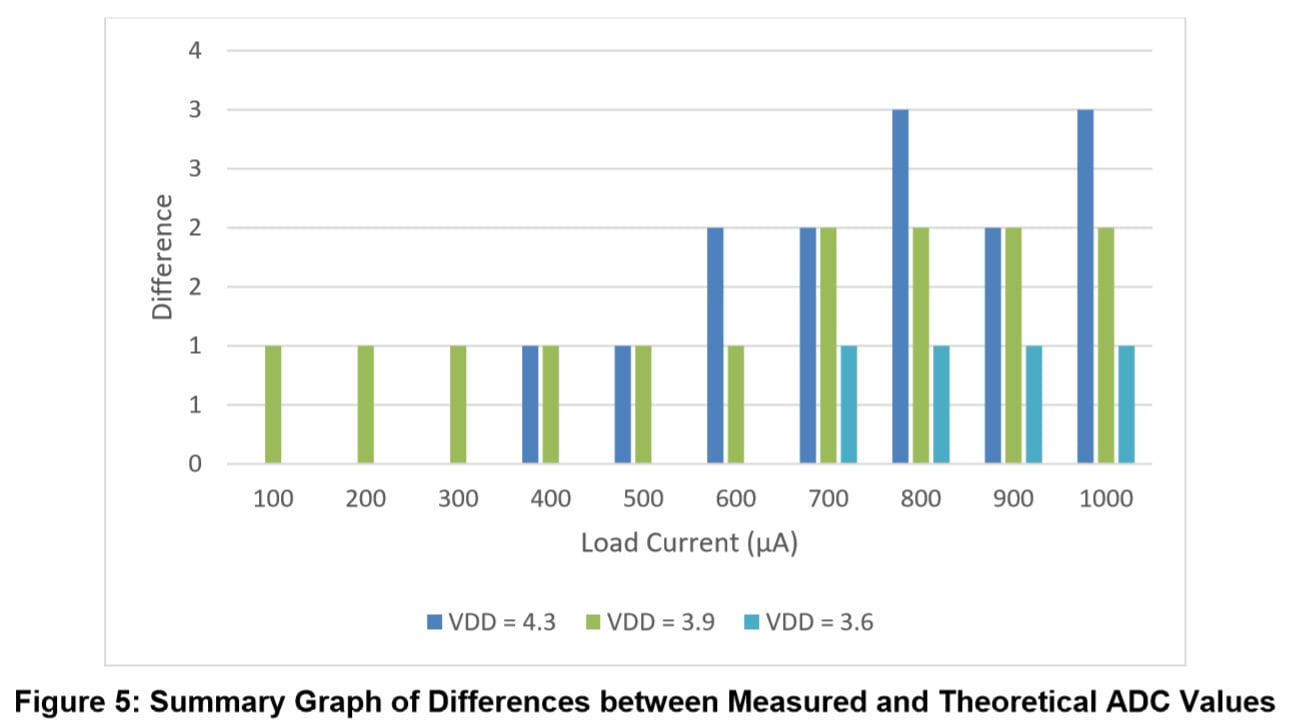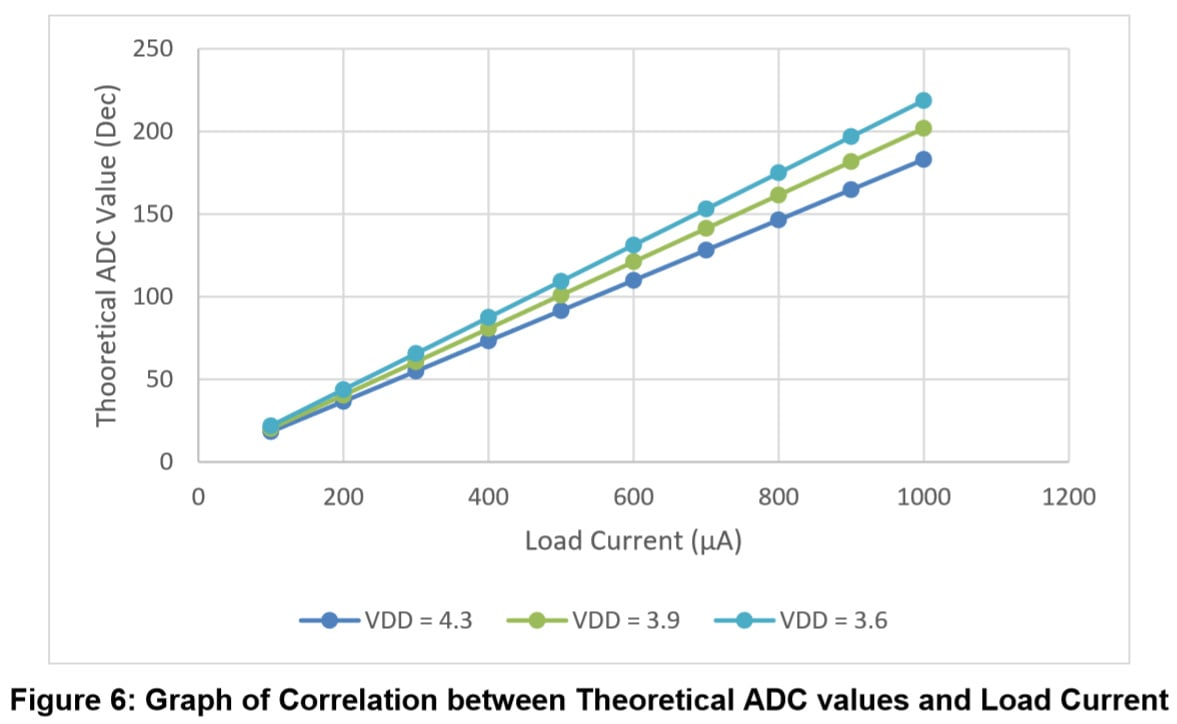## Conclusions

The device was tested at three voltage levels: 3.6 V, 3.9 V, and 4.3 V. The range of these voltages models a full lithium-ion battery that discharges to its nominal level. Of the three voltage levels, it is observed that the device typically was more accurate at 3.9 V for the chosen external circuit. The difference between the measured and theoretical ADC values was only 1 decimal value off at load currents of 700 - 1000 ÂµA. At the given voltage range, the measured ADC values were 3 decimal points above nominal conditions at the worst case. Further adjustments to the resistor divider can be made to optimize different VDD voltage levels.